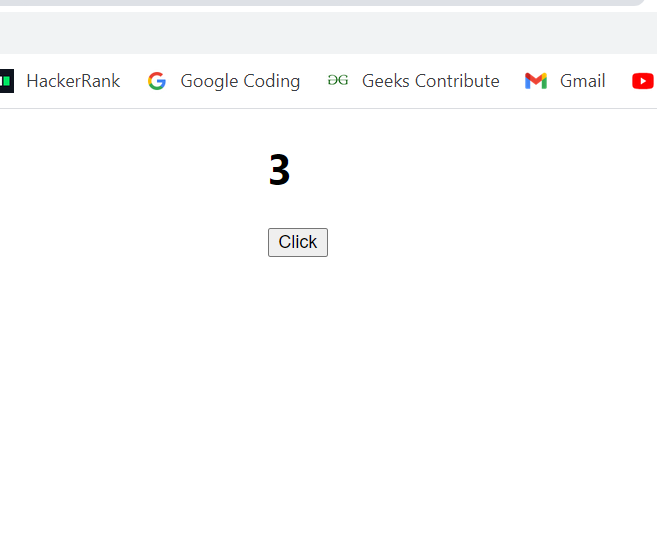# What is useState() in React ?

• Last Updated : 09 Jun, 2022

The useState() is a Hook that allows you to have state variables in functional components . so basically useState is the ability to encapsulate local state in a functional component. React has two types of components, one is class components which are ES6 classes that extend from React and the other is functional components. Class components a Component and can have state and lifecycle methods: class Message extends React. The  useState hook is a special function that takes the initial state as an argument and returns an array of two entries.  UseState encapsulate only singular value from the state, for multiple state need to have useState calls.

Syntax: The first element is the initial state and the second one is a function that is used for updating the state.

`const [state, setState] = useState(initialstate)`

We can also pass a function as an argument if the initial state has to be computed. And the value returned by the function will be used as the initial state.

`const [sum, setsum] = useState(function generateRandomInteger(){5+7);})`

The above function is oneline function which computes the sum of two numbers and will be set as the initial state.

Importing: To use useState you need to import useState from react as shown below:

`import React, { useState } from "react"`

Creating React Application:

Step 1: Create a React application using the following command:

`npx create-react-app foldername`

Step 2: After creating your project folder i.e. foldername, move to it using the following command:

`cd foldername`

Example: Below is the implementation of useState() function.

App.js

## Javascript

 `import React, { useState } from ``"react"``;` `function` `App(props) {``  ``const [count, setRandomCount] =``    ``useState(``function` `generateRandomInteger() {``    ``return` `Math.floor(Math.random() * 100);``  ``});``  ``function` `clickHandler(e) {``    ``setRandomCount(Math.floor(Math.random() * 100));``  ``}``  ``return` `(``    ``
``      ``

{count}

``      ` `      ` `

``        ````      ``

`  `    ``
``  ``);``}` `export ``default` `App`

Step to Run Application: Run the application using the following command from the root directory of the project:

`npm start`

Output:Explanation: First count variable is initialized with a random number using a random function and setRandomCount is used for updating the state of the count. Every time we click on the button onClick it calls the clickHandler function which sets the count variable again with a random number.

My Personal Notes arrow_drop_up